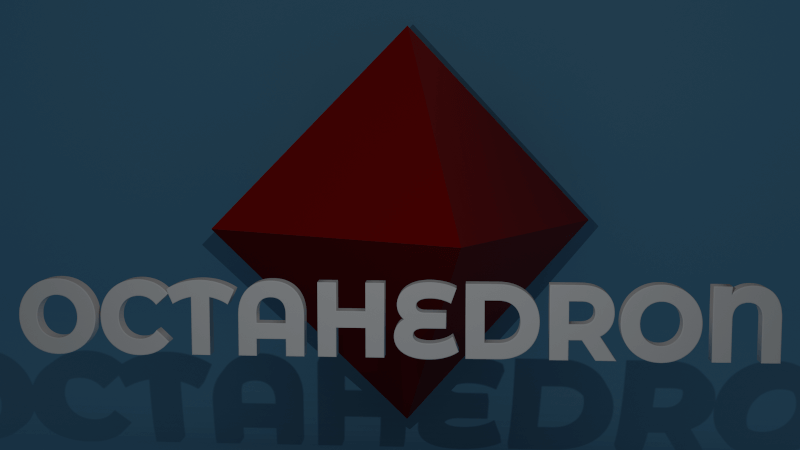# Octahedrons and how to draw their net

Subject: tutorials

Description: What is a Octahedron and how to draw it's net

Posted by David Caldwell on 07/04/20 at 20:02

## What is an Octahedron?An Octahedron is a three-dimensional shape with eight faces.   The word Octahedron is Greek and comes from ‘Octa’ meaning eight and ‘hedron’ meaning sided.

An Octahedron can be thought of two square-based pyramids joined together by the base.

Each face of a regular Octahedron is an equilateral triangle.

An equilateral triangle is a triangle that has three sides the same length and have the same angle in each corner.

As well as having eight faces an Octahedron has twelve edges and six corners.   In geometry another name for corner is vertices.

Faces in an Octahedron are parallel to their opposite. Which means you can use an Octahedron for a table.  They are also a good shape for dice as they always land with one face up.

If you are a fan of role-playing games you probably know about an octahedron known as a D8 or eight-sided dice.

In nature, octahedra can be found in crystals and gems such as diamonds.

An Octahedron is one of the five platonic solids.

## Platonic Solids

Plato lived two and a half thousand years ago in the city of Athens in Greece.  He was a philosopher and mathematician and taught students in his own school called the Akademia.  He must have liked symmetry because he died on the same day of the year that he was born.

In ancient times they didn’t have the periodic table and believed that there were just four elements.

These were earth, air, wind and fire.  Plato connected a 3D shape or solid with each element.

• A cube represents earth.
• Our octahedron represents air.
• An Icosahedron represents water
• A tetrahedron represents fire.

Plato had one shape left over.  This was the mighty dodecahedron which Plato connected to the mysterious element which is space itself, the emptiness which joins all the other elements together.

## What is Duality

Platonic solids have an exciting property.  They share a duality with another solid.  The tetrahedron is the odd one out as it shares a duality with itself.

Can you guess which solid the octahedron has a duality with?

Imagine you draw a dot in the centre of each face.  Join the dots together with lines through the octahedron’s interior and what shape do you get?  The answer is a cube.

The strange thing is that if you did the same with a cube you would get an octahedron.

Mind-blowing.  Right?

### Step by Step Guide to Drawing an Octahedron Net

• Draw a horizontal line just below the centre of the page.
• In the middle of the line draw a circle.
• Draw two more circles on either side of the first using the points where the line intersects with the first circle.
• Draw two more circles using the points where the three circles intersect above the line.
• Draw an arc where the line leaves the far left circle.
• Draw an arc where the top left circle intersects the bottom left circle.
• Create eight equilateral triangles by joining the intersections as above.
• If cutting out to make a model.  Create tabs for glue.
• Using a fine-liner and ruler ink in your lines and erase construction lines.
• The finished net is ready to cut out and construct.

### Step by Step Guide to Drawing a Square Based Pyramid

• Draw a horizontal line.
• Placing the compass at each end of the line and create an arc above and below to create intersections.
• Draw a vertical line through the two intersections.
• Draw a circle using where the lines cross as the central point.